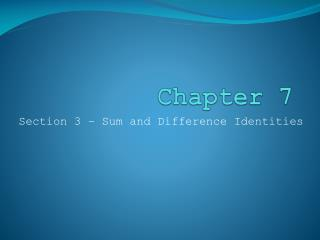# Chapter 7 - PowerPoint PPT PresentationDownload PresentationChapter 7

Chapter 7
Download Presentation## Chapter 7

- - - - - - - - - - - - - - - - - - - - - - - - - - - E N D - - - - - - - - - - - - - - - - - - - - - - - - - - -
##### Presentation Transcript

1. Chapter 7 Section 3 – Sum and Difference Identities

2. Sum/Difference of Cosine • Derivation – to find the length of a segment between 2 points on a Unit Circle formed by 2 angles, you can solve using Law of Cosines • Which gives: 2 – 2cos(a – b) Or by using the distance formula, which gives: 2 – 2(cos(a)cos(b)+sin(a)sin(b) So these two must be equal! 2-2cos(a-b) = 2-2(cos(a)cos(b)+sin(a)sin(b) So… cos(a-b) = cos(A)cos(b) + sin(a)sin(b)

3. Sum/Difference of Cosine • Given: cos(a-b) = cos(A)cos(b) + sin(a)sin(b) Then Likewise, the sum cos(a+b) (can get the same way, just replacing b with –b) cos(a+b) = cos(a)cos(b) – sin(a)sin(b)

4. Sum/Difference of Sine • Using the phase shift difference between sine and cosine, and the previous identity, we can derive sum/difference of sine function • Sin(a±b)=sin(a)cos(b)± cos(a)sin(b)

5. Sum/Difference of Tan • Plug sin and cos identities into ratio of Tangent. You get: • Tan(a±b) =

6. Sum/Difference Identities • You can use these identities along with your knowledge of special angles to find other angles derived from them!

7. Assignment • Chapter 7, Section 3 pgs442 #14 – 22 even